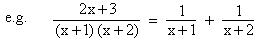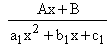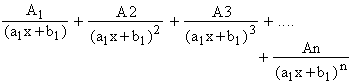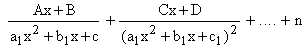Home MonkeyNotes Printable Notes Digital Library Study Guides Study Smart Parents Tips College Planning Test Prep Fun Zone Help / FAQ How to Cite New Title Request

6.7 Integration By Partial Fractions

If an algebraic fraction is expressed in terms of two or more simpler fractions, then this simple fractions are called partial fractions.In many problems, if we resolve an algebraic fraction into partial fractions, its integration becomes much simpler.

Let the fraction to be resolved into partial fraction is. Then there are two cases

(1) Degree of P (x) < degree of Q (x).

This algebraic fraction is known as the proper fraction.

(2) Degree of P(x) > degree of Q (x).

This type of algebraic fraction is known as an improper fraction.

Consider these cases :

(a) To each linear factor (a1x2 + b1) of Q (x), there will be a corresponding partial fraction in the form(b) To each quadratic factor (a1x2 + b1x + c1), there will be a corresponding partial fraction in the form(c) To each repeated linear factor (a1x + b1)n of Q (x), there will be n corresponding partial fractions of the formYour browser does not support the IFRAME tag.

(d) To each repeated Quadratic factor (a1x2 + b1 + c1 )n there will be corresponding  n  partial fractions astermsIndex

6.1 Anti-derivatives (indefinite Integral)
6.2 Integration of Some Trigonometric Functions
6.3 Methods of Integration
6.4 Substitution and change of variables
6.5 Some Standard Substitutions
6.6 Integration by Parts 6.7 Integration by Partial Extractions

Chapter 7